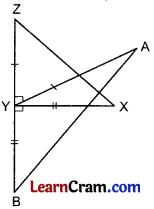# DAV Class 7 Maths Chapter 9 Brain Teasers Solutions

The DAV Maths Class 7 Solutions and DAV Class 7 Maths Chapter 9 Brain Teasers Solutions of Construction of Triangles offer comprehensive answers to textbook questions.

## DAV Class 7 Maths Ch 9 Brain Teasers Solutions

Question 1.
A. Tick (✓) the correct option:
(i) If ΔABC ≅ ΔPQR, which of the following is not true?
(a) ∠A = ∠P
(b) BC = QR
(c) ∠B = ∠R
(d) AC = PR
(c) ∠B = ∠R

ΔABC and ΔPQR are two congruent triangles. Superimpose AABC on APQR so as to cover it exactly. In such a superimposed condition, the vertices of AABC will fall on the vertices of APQR in same order.So, vertex A falls on vertex P, vertex B falls on vertex Q, and vertex C falls on vertex R, then side AB will fall on side PQ, BC on QR and CA on RP. Also, ZA will superimpose on the corresponding angle ∠P, ∠B on ∠Q and ∠C on ∠R. Therefore, ∠B = ∠R is not true.
Hence (c) is the correct option.

(ii) Which of the following criterion is not true for the congruence of two triangles?
(a) SAS
(b) SSS
(c) SSA
(d) ASA
(c) SSA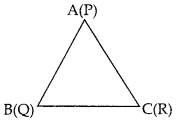SSA is not any criterion for the congruence of two triangles.
Hence, (c) is the correct option.

(iii) If ΔART ≅ ΔPEN, which of the following is true?
(a) AR = PE
(b) ∠R = ∠N
(c) AR = PN
(d) ∠T = ∠P
ΔART and ΔPEN are two congruent triangles. Superimpose ΔART on ΔPEN so as to cover it exactly. In such a superimposed condition, the vertices of ΔART will fall on the vertices of ΔPEN in some order. Vertex A falls on vertex P, vertex R falls on vertex E, and vertex T falls on R vertex N, then side AR will fall on side PE, RT on EN and TA on NP. Also, ∠A will superimpose on the corresponding angle ∠P, ∠R on ∠E and ∠T on ∠N.∴ AR = PE is true.
Hence, (a) is the correct option.

(iv) Which of the following criterion will you use if ΔABC ≅ ΔDEF and AB = DE, ∠B = ∠E, ∠C = ∠F,
(a) ASA
(b) SSS
(c) SAS
(d) RHS
In ΔABC and ΔDEF, we haveAB = DE
∠B = ∠E
∠C = ∠F
So, in ΔABC and ΔDEF, the two angles and any one side are equal to the corresponding two B‘ angles and one side of the other triangle.
Therefore, the ΔABC and ΔDEF are congruent. So the condition AAS leads to ASA.
Hence, (i) is the correct option.

(v) In the given figure equal side are marked. Which of the following A triangles are congruent?
(c) ΔBDA ≅ ΔBDC
(d) ΔDAB ≅ ΔDCB
In the given figure,AB = DC [Given]
BD = BD [Common side]
Now, superimpose ΔCBD on ΔADB so as to cover it exactly with C on A, B on D and D on B. So, we find that the two triangles cover each other exactly.
Therefore, we can conclude that ΔADB ≅ ΔCBD.(i) When are two circles said to be congruent?
Two circles are congruent, if they have the same radii.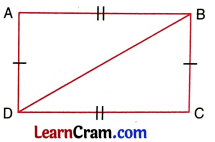In the above figure,
P = Q, since P covers Q exactly (the two circles have equal radius).

(ii) What is the side included between the angles M and N of ∆MNP?
In the given figure, MN is the side included between the angles M and N of ∆MNP.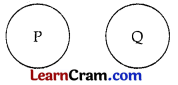(iii) If ∆ABC and ∆PQR are to be congruent, name one additional pair of corresponding part to make them congruent by ASA condition.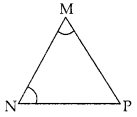Superimpose ∆ABC over ∆PQR.
We will observe that with A on P, B on Q and C on R, ∆ABC covers ∆PQR exactly, or the matching ABC ↔ PQR gives an exact cover of the two triangles by each other.Now, in the two triangle ABC and PQR, the pairs of corresponding parts are:
∠B = ∠Q [Given]
∠C = ∠R [Given]
For ASA congruence condition, the third pair of corresponding parts which should be equal, are the sides BC and QR of the two triangles.
Thus, the third pair of corresponding parts to make ∆ABC ≅ ∆PQR by ASA congruence condition are :
BC = QR

(iv) In the given figure, the two triangles are congruent. The corresponding parts are marked. Complete ∆RAT =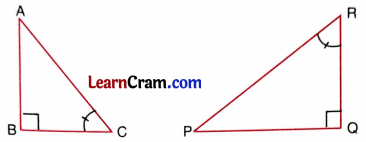From the figures, we note that ∠A = ∠O, ∠R = ∠W and ∠T = ∠N.
Also, AT = NO and RA = WO, therefore, RT = WN.

Thus, the correspondence RAT ↔ WON gives a congruence.
Hence, ∆RAT ≅ ∆WON

(v) ABCD is a rectangle, BD is the diagonal of the rectangle. Which all congruence creterion can be used to show ∆ABD ≅ ∆DCB?
In ∆ABD and ∆DCB,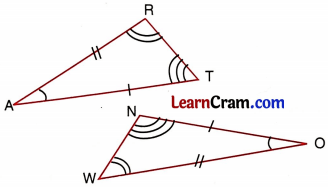AB = DC [∵ Opposite sides of a rectangle are equal]
BC = AD [∵ Opposite sides of a rectangle are equal]
BD = BD [Common side]
So, by SSS congruence condition, we have ∆ABD ≅ ∆DCB
Again, in right triangles ABD and DCB,
∠BAD = ∠BCD = 90° [Angles of a rectangle]
BD = BD [Common hypotenuse]
AD = BC [∵ Opposite sides of a rectangle are equal]
Therefore, by RHS congruence condition,
∆ABD ≅ ∆DCB
So, SSS and RHS congruence criterions can be used to show ∆ABD ≅ ∆DCB.Question 2.
In the given figure, each pair of triangles has similar markings indicating equal parts. Specify, by which condition, the two triangles will become congruent. Write the result in symbolic form.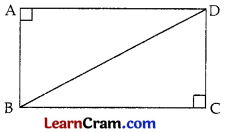In ∆AOB and ∆COD,
OA = OC (given)
OB = OD (given)
∠AOB = ∠COD (Vertically opposite angles)
∴ ∆AOB ≅ ∆COD (By SAS)In ∆ABD and ∆ACD,
AB = AC (given)
BD = CD (given)
∴ ∆ABD ≅ ∆ACD (By SSS)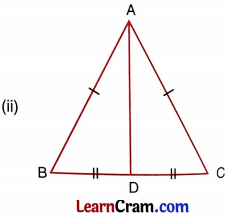In ∆ABD and ∆ACD,
AB = AC (given)
BD = CD (given)
∴ ∆ABD ≅ ∆ACD (By SSS)In ∆ABC and ∆QRP,
∠B = ∠R (given)
∠C = ∠P (given)
BC = PR (given)
∴ ∆ABC ≅ ∆QRP (ASA)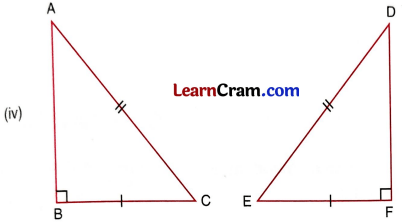In ∆ABC and ∆DFE,
AC = DE (given)
BC = FE (given)
∠B = ∠F = 90° (given)
∴ ∆ABC ≅ ∆DFE (By RHS)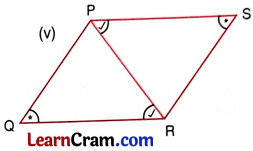In ∆PQR and ∆RSP,
∠Q = ∠S (given)
∠QRP = ∠SPR (given)
∠QPR = ∠SRP (Remaining III angle)
PR = PR (Common)
∴ ∆PQR ≅ ∆RSP (By ASA)

Question 3.
Fill in the blanks, so as to make the following statements true.
(i) If PQ = YZ, ∠Q = ∠Z and QR = ZX, then ∆PQR = by SAS congruence condition.
∆YZX

(ii) If ∆ABC ≅ ∆EFD then side BC = side and ∠A =
FD, ZE

(iii) If in two triangles ABC and DEF, ∠A = ∠D = 90°, hypotenuse BC = hypotenuse EF, side AB = side DE, then ∆ABC ≅ ∆DEF by congruence condition.
RHSQuestion 4.
Show that in an isosceles triangle, angles opposite to equal sides are equal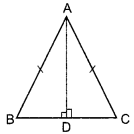In ∆ABD and ∆ACD,
AB = AC (given)
∆ABD ≅ ∆ACD (By RHS)
∠ABD = ∠ACD (C.P.C.T.)
Hence Proved.

Question 5.
In the given figure, P and Q are two points on equal sides AB and AC of an isosceles triangle ABC such that AP = AQ. Prove that BQ = CPIn ∆APC and ∆AQB,
AP = AQ [given]
AC = AB (given)
∠A= ∠A
∆APC ≅ ∆AQB
∴ BQ = CP

Question 6.
In the given figure, ABC is an isosceles triangle in which AB = AC.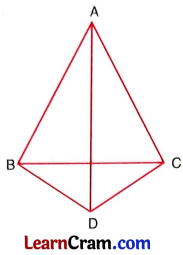Also, D is a point such that BD = CD. Prove that AD bisects ZA and ZD.
In ∆ABD and ∆ACD,
AB = AC (given)
BD = DC (given)
∴ ∆ABD ≅ ∆ACD (SSS)
Hence AD bisects ∠A and ∠D.

Question 7.
In the given figure, PQR and SQR are two triangles on a common base QR such that PQ = SR and PR = SQ.(i) Is ∆PSQ ≅ ∆SPR? By which congruence condition?
In ∆PSQ and ∆SPR,
PQ = SR
PR = SQ
PS = PS
∴ ∆PSQ ≅ ∆SPR

(ii) State the three pairs of corresponding parts you have used to answer (i).
PQ and SR, PR and SQ, PS and PS

(iii) If ∠SRP = 40° and ∠QPS = 110°, then find ∠PSQ.
∠SRP = ∠PQS = 40°
∠QPS = 110°
∠PQS + ∠QPS + ∠PSQ = 180°
⇒ 40° + 110° + ∠PSQ = 180°
⇒ 150° + ∠PSQ = 180°
∠PSQ = 180° -150° = 30°
Hence ∠PSQ = 30°.

## DAV Class 7 Maths Chapter 9 HOTS

Question 1.
In the given figure, AD = AE, BD = CE. Prove that ∆AEB ≅ ∆ADC.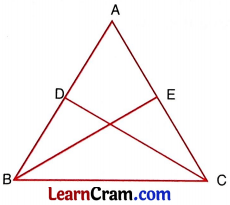In the given figure,
BD = CE …(2)
Adding equations (1) and (2), we get
AD + BD = AE + CE
⇒ AB = AC

Since two sides of ∆ABC are equal i.e., AB = AC
So ∠B = ∠C [ Y Angles opposite to equal sides of a triangle are equal]
Now, in ∆DBC and ∆ECB, we have
BD = CE
BC = BC
∠B = ∠C

So, by SAS congruence condition, we have
∆DBC = ∆ECB
BE = CD [Corresponding parts of congruent triangles]
Now, in ∆AEB and ∆ADC, we have
AB = AC [Proved above]
BE = CD [Proved above]
Therefore, by SSS congruence condition, we have
Hence, proved.

Question 2.
In the given figure, AB = AC, BD = EC. Prove that ∆ABE ≅ ∆ACD and AD = AE.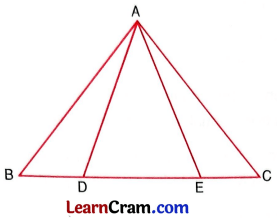In ∆ABC, we have
AB = AC
∴ ∠B = ∠C
[∵ Angles opposite to equal sides of a triangle are equal]
Now, in ∆ABD and ∆AEC, we have B D E
AB = AC [Given]
BD = EC
∠B = ∠C
Therefore, by SAS congruence condition, we have
∆ABD ≅ ∆AEC
⇒ ∠D = ∠E
Now, in ∆ABE and ∆ACD, we have
AB = AC
∠B = ∠C
∠E = ∠D
Therefore, by ASA congruence condition, we have
∆ABE ≅ ∆ACD
Hence, proved.

## DAV Class 7 Maths Chapter 9 Enrichment Questions

Question 1.
Prove that the bisector of the vertical angle of an isosceles triangle is perpendicular to the base.
Let ∆ABC be an isoceles triangle such that AB = AC and AD is the bisector of ∠A meeting BC in D.
In ∆ABD and ∆ACD, we have
AB = AC [Given]
Therefore, by SAS congruence condition, we have
∆ABD ≅ ∆ACD [Corresponding parts of congruent triangles]
⇒ ∠ADB = $$\frac{180^{\circ}}{2}$$
Hence, AD bisects BC at right angles.

Question 2.
In the given figure, AE = DE, CE = BE, then prove that AB = CD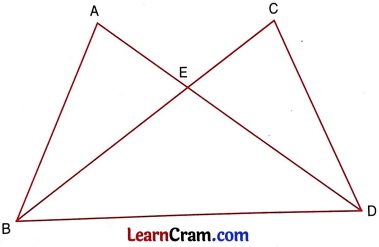In ∆ABE and in ∆CDE, we have
AE = ED [Given]
CE = EB [Given]
∠AEB = ∠CED [Vertically opposite angles]
Therefore, by SAS congruence condition, we have ∆ABE ≅ ∠DCE
∴ AB = CD [Corresponding parts of congruent triangles]

Question 1.
From the given figures, state which pair of triangles are congruent and write them in symbolic form.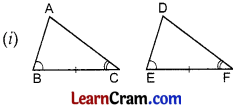∠B = ∠E (given)
∠C = ∠F (given)
BC = EF (given)
∴ ∆ABC ≅ ∆DEF (By ASA)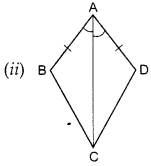AC = AC (Common)
∠BAC = ∠DAC (given)AB = CD (given)
∠B = ∠D = 90° (given)
BO = OD (given)
∴ ∆ABO ≅ ∆CDO (RSH)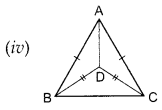AB = AC (given)
BD = CD (given)
∴ ∆ABD ≅ ∆ACD (SSS)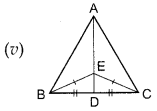BE = CE (given)
BD = CD (given)
ED = ED (common)
∴ ∆EBD ≅ ∆ECD (SSS)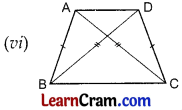AB = CD (given)
BC = BC (common)
∴ ∆ABC ≅ ∆DBC (SSS)

Question 2.
Prove that the median of an isosceles triangle divides the triangle into two congruent triangles.
AD is the median of ∆ABC such that BD = DC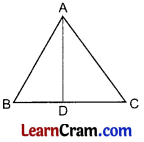In ∆ABD and ∆ACD,
AB = AC (given)
BD = DC (given)
∆ABD ≅ ∆ACD (By SSS)Question 3.
In the given figure, BA = CA. They are respectively produced to Q and P such that AP = AQ. Prove that PB = QC.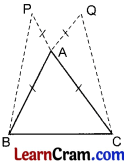In ∆APB and ∆AQC,
AB = AC (given)
AP = AQ (given)
∠PAB = ∠QAC (Vertically opposite angles)
∆APB ≅ ∆AQC
PB = QC (BY C.P.C.T)

Question 4.
In the given figure, M is the mid point of AB and CD. Prove that CA = BD and CA ∥ BD.In ∆AMC and ∆BMD
AM = BM (given)
CM = DM (given)
∠AMC = ∠BMD (Vertically opposite angles)
∆AMC ≅ ∆BMD (SAS)
CA = BD (C.P.C.T)
and ∠CAM = ∠DBM (C.P.C.T)

Question 5.
In the given figure, AY ⊥ ZY and BY ⊥ XY such that AY = AY and BY = XY. Prove that AB = ZX.